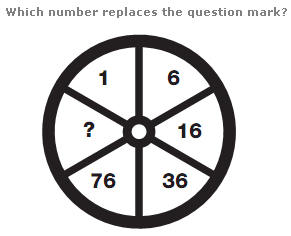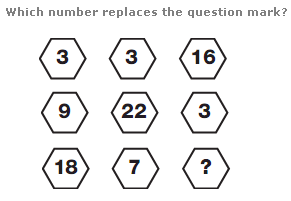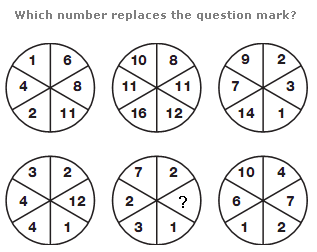# Puzzles - Number puzzles

### Exercise :: Number puzzlesAnswer : 156 Explanation : Start with 1 and move clockwise. Multiply the previous number by 2 and add 4 to give the next number around.Answer : 1 Explanation : In every row of the diagram, if you subtract the odd numbers from the even number, you always get 10.Answer : 5 Explanation : The values in the segments of the upper middle circle equal the sum of the values in corresponding segments of the upper left and right hand circles, and the values in the segments of the lower middle circle equal the difference between values in corresponding segments of the lower left and right hand circles.